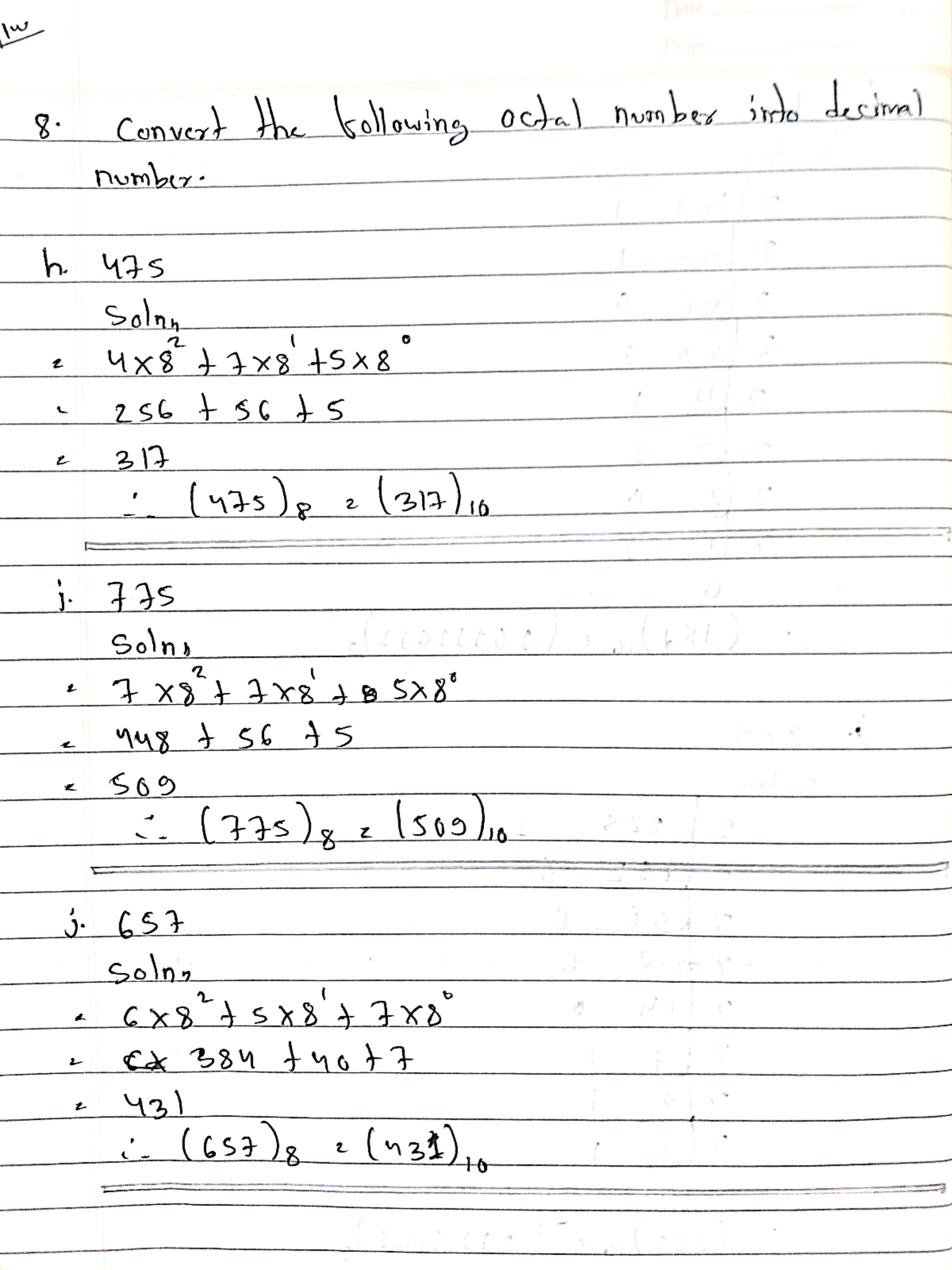# Number System: Class 10 Computer Exercise - New Syllabus

## Chapter -  7   Number System

📚EXERCISES

### 1. Answer the following questions:

a. What is a number system? List the different types of number system.

Ans: Number System is the System used to represent or express numbers. The different types of number system are as follows:

• Decimal number system (Base- 10)
• Binary number system (Base- 2)
• Octal number system (Base- 8)
• Hexadecimal number system (Base- 16)

b. What is binary number system?

Ans: The number system that uses two different digits i.e 0 and 1 is known as binary number system.

c. What is hexadecimal number system? List its digits.

Ans: The number system that uses 16 different digits to represent number is known as hexadecimal number system. It's digits are 0, 1, 2, 3, 4, 5, 6, 7, 8, 9, A, B, C, D, E, and F

d. What is octal number system? List its digits.

Ans: The number system that uses 8 different digits to represent number is known as octal number system. It's digits are 0, 1, 2, 3, 4, 5, 6 and 7.

e. What are BIT and Byte.

Ans: BIT is defined as the smallest unit of data in a computer.

Byte is defined as a group of binary digits or bits (usually eight) operated on as a unit.

## 2. Perform the following Binary Calculations.